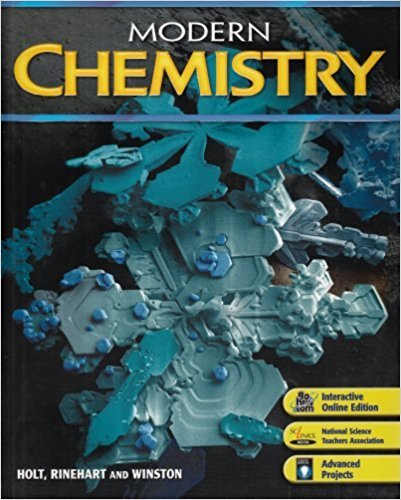×
×

# Under what circumstances might we prefer to express solution concentrations in terms ofISBN: 9780030735462 452

## Solution for problem 17 Chapter 12

Modern Chemistry | 1st Edition

• Textbook Solutions
• 2901 Step-by-step solutions solved by professors and subject experts
• Get 24/7 help from StudySoup virtual teaching assistantsModern Chemistry | 1st Edition

4 5 1 339 Reviews
22
0
Problem 17

Under what circumstances might we prefer to express solution concentrations in terms of a. molarity? b. molality?

Step-by-Step Solution:
Step 1 of 3

Naming compounds l Binary compounds: compounds composed of two elements l Binary ionic compound: contain a positive ion (cation), which is always written first in the formula, and a negative ion (anion) l In the formulas of ionic compounds, simple ions are represented by the element symbol but when individual...

Step 2 of 3

Step 3 of 3

##### ISBN: 9780030735462

Since the solution to 17 from 12 chapter was answered, more than 298 students have viewed the full step-by-step answer. Modern Chemistry was written by and is associated to the ISBN: 9780030735462. This full solution covers the following key subjects: . This expansive textbook survival guide covers 23 chapters, and 1171 solutions. The answer to “Under what circumstances might we prefer to express solution concentrations in terms of a. molarity? b. molality?” is broken down into a number of easy to follow steps, and 17 words. The full step-by-step solution to problem: 17 from chapter: 12 was answered by , our top Chemistry solution expert on 03/16/18, 03:02PM. This textbook survival guide was created for the textbook: Modern Chemistry, edition: 1.

Unlock Textbook Solution